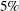The GENMOD Procedure

Example 43.12 Tweedie Regression

The following SAS statements simulate 250 observations, which are based on an underlying Tweedie generalized linear model (GLM) that exploits its connection with the compound Poisson distribution. A natural logarithm link function is assumed for modeling the response variable (yTweedie), and there are five categorical variables (C1C5), each of which has four numerical levels and two continuous variables (D1 and D2). By design, two of the categorical variables, C3 and C4, and one of the two continuous variables, D2, have no effect on the response. The dispersion parameter is set to 0.5, and the power parameter is set to 1.5.

%let nObs = 250;
%let nClass = 5;
%let nLevs = 4;
%let seed = 100;

data tmp1;
array c{&nClass};

keep c1-c&nClass yTweedie d1 d2;

/* Tweedie parms */
phi=0.5;
p=1.5;

do i=1 to &nObs;

do j=1 to &nClass;
c{j} = int(ranuni(1)*&nLevs);
end;

d1 = ranuni(&seed);
d2 = ranuni(&seed);

xBeta   =  0.5*((c2<2) - 2*(c1=1) + 0.5*c&nClass + 0.05*d1);
mu      =  exp(xBeta);

/* Poisson distributions parms */
lambda = mu**(2-p)/(phi*(2-p));
/* Gamma distribution parms */
alpha = (2-p)/(p-1);
gamma = phi*(p-1)*(mu**(p-1));

rpoi = ranpoi(&seed,lambda);
if rpoi=0 then yTweedie=0;
else do;
yTweedie=0;
do j=1 to rpoi;
yTweedie = yTweedie + rangam(&seed,alpha);
end;
yTweedie = yTweedie * gamma;
end;
output;
end;
run;


The following SAS statements invoke PROC GENMOD to fit the Tweedie GLM with the log link using all of the categorical and continuous variables. A Type III analysis is requested by the TYPE3 option in the MODEL statement.

proc genmod data=tmp1;
class C1-C5;
model yTweedie = C1-C5 D1 D2 / dist=Tweedie type3;
run;


The "Criteria For Assessing Goodness Of Fit" table is displayed in Output 43.12.1. The scaled Pearsonis close to 1, indicating that the specified model fits the data well.

Output 43.12.1: Tweedie Goodness of Fit Criteria

The GENMOD Procedure

Criteria For Assessing Goodness Of Fit
Criterion DF Value Value/DF
Pearson Chi-Square 232 101.9124 0.4393
Scaled Pearson X2 232 251.5826 1.0844
Log Likelihood   -297.2106
Full Log Likelihood   -297.2106
AIC (smaller is better)   634.4212
AICC (smaller is better)   638.0893
BIC (smaller is better)   704.8504

The "LR Statistics For Type 3 Analysis" table is displayed in Output 43.12.2. As expected, the p-values for C3, C4, and d2 are not statistically significant at thelevel.

Output 43.12.2: Type III Analysis of Covariate Effects

LR Statistics For Type 3 Analysis
Source DF Chi-Square Pr > ChiSq
c1 3 85.46 <.0001
c2 3 48.18 <.0001
c3 3 0.56 0.9050
c4 3 9.38 0.0247
c5 3 47.76 <.0001
d1 1 0.00 0.9595
d2 1 1.31 0.2518

You can fix the power parameter for fitting the Tweedie GLM by using the P= option. The following SAS statements fit the model for C1, C2 and D1, while holding the power parameter at 1.5:

proc genmod data=tmp1;
class C1 C2;
model yTweedie = C1 C2 D1 / dist=Tweedie(p=1.5) type3;
run;


The parameter estimates are displayed in Output 43.12.3.

Output 43.12.3: Tweedie Maximum Likelihood Parameter Estimates

The GENMOD Procedure

Analysis Of Maximum Likelihood Parameter Estimates
Parameter   DF Estimate Standard
Error
Wald 95% Confidence Limits Wald Chi-Square Pr > ChiSq
Intercept   1 0.3440 0.1347 0.0801 0.6080 6.53 0.0106
c1 0 1 -0.0722 0.1101 -0.2880 0.1436 0.43 0.5120
c1 1 1 -0.8952 0.1196 -1.1296 -0.6607 56.01 <.0001
c1 2 1 0.0770 0.1073 -0.1334 0.2873 0.51 0.4733
c1 3 0 0.0000 0.0000 0.0000 0.0000 . .
c2 0 1 0.6138 0.1161 0.3862 0.8414 27.93 <.0001
c2 1 1 0.5103 0.1150 0.2849 0.7356 19.70 <.0001
c2 2 1 0.1001 0.1215 -0.1380 0.3381 0.68 0.4099
c2 3 0 0.0000 0.0000 0.0000 0.0000 . .
d1   1 -0.0211 0.1493 -0.3136 0.2714 0.02 0.8876
Dispersion   1 0.4951 0.0398 0.4172 0.5731
Power   0 1.5000 0.0000 1.5000 1.5000

 Note: The Tweedie dispersion parameter was estimated by maximum likelihood. Note: The Tweedie power parameter was held fixed.